Related Articles

# Parallel Lines and Transversals

• Last Updated : 30 Apr, 2021

You’ve probably seen a set of train lines, a staircase, or a set of piano keys. Is there something that any of these have in common? The two tracks never cross, and the ladder’s two sides never meet. The piano keys are parallel to each other at all times. Let’s take a closer look at parallel and transversal lines, as well as the angles that they correspond to.

Parallel lines: The combination of two or more lines that are stretched to infinity and never intersect each other are called the parallel line or coplanar lines. The parallel lines are denoted by a special symbol, given by ||.

Transversal: A transversal of any given line is a line that intersects two or more given lines at distinct points.

Corresponding angles: The angles made when a transversal intersect with any pair of parallel lines are called the corresponding angles.

The figure below shows both parallel and transversal lines along with the corresponding angles formed by them as: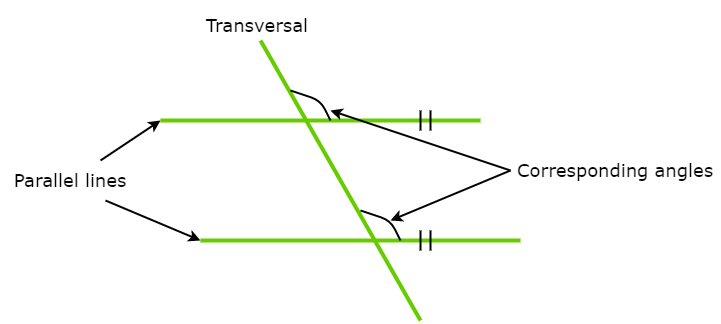### Parallel lines and a transversal

Consider a line l that intersects lines m and n at points P and Q respectively. Therefore, line l is a transversal for lines m and n where eight different angles are obtained. The eight angles together form four pairs of corresponding angles. As observed in the figure below, ∠2 and ∠6 constitute a pair of corresponding angles. All angles that have the same position with respect to the lines and the transversal are the pair of corresponding angles.

Moreover, the angles that are in the area between the lines e.g. ∠4 and ∠5 are called interior angles whereas the angles that are on the outer side of the two lines e.g. ∠1 and ∠8 are called exterior angles. The angles that are on the opposite sides of the transversal are called alternate angles e.g. ∠4 and ∠6. The angles which share the same vertex and have a common ray, e.g. angles ∠1 and ∠2 or ∠6 and ∠5 in the figure are called adjacent angles. In this case where the adjacent angles are formed by two lines intersecting two pairs of adjacent angles that are supplementary are obtained. The two angles that are opposite to each other as ∠1 and ∠3 in the figure are called vertical angles.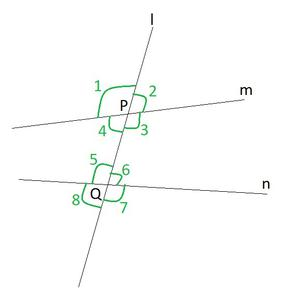Angles made by Parallel lines and a transversal

Hence, the pair of corresponding angles, alternate interior angles, alternate exterior angles, Interior angles on the same side of the transversal are as follows:

• Corresponding angles: ∠ 1 and ∠ 5, ∠ 2 and ∠ 6, ∠ 4 and ∠ 8 and ∠ 3 and ∠ 7.
• Alternate interior angles: ∠ 4 and ∠ 6, and ∠ 3 and ∠ 5.
• Alternate exterior angles: ∠ 1 and ∠ 7, and ∠ 2 and ∠ 8.
• Interior angles on the same side of the transversal: ∠ 4 and ∠ 5, and ∠ 3 and ∠ 6.

### Corresponding Angles Axiom

Let us find out the relation between the angles in these pairs when line m is parallel to line n.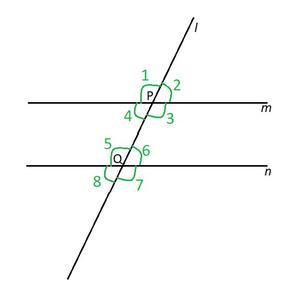Pair of Corresponding Angles.

Therefore, the Corresponding Angles Axiom is stated as:

If a transversal intersects two parallel lines, such that a pair of corresponding angles is equal, then the two lines are parallel to each other.

To Prove: Corresponding angles are equal.

Proof: Line m and n are parallel to each other and line l is traversal.

Since line m and n are parallel.

Therefore,

∠ 3 + ∠ 6 = 180°                                      (Adjacent angle of parallelogram)               ……(1)

∠ 7 + ∠ 6 = 180°                                      (Supplementary angles)                               ……(2)

∠ 3 + ∠ 2 = 180°                                      (Supplementary angle)                                ……(3)

So, from equation (1) and (2) it is concluded that:

∠ 3 = ∠ 7

Similarly, from (1) and (3) it is concluded that:

∠ 6 = ∠ 2

In this way, it is also can be proved that:

∠ 1 = ∠ 5

∠ 4 = ∠ 8

This implies, that all the four pairs of corresponding angles are equal to each other.

Converse of Corresponding Angles Axiom

The converse of corresponding angles axiom is stated as:

If a transversal intersects two lines such that a pair of corresponding angles is equal, then the two lines are parallel to each other.Pair of Corresponding Angles.

To Prove: If corresponding angles are equal, then lines are parallel.

Proof: Line l is traversal to lines m and n.

Therefore,

∠ 3 = ∠ 7                             (Given, corresponding angles are equal)                ……(1)

∠ 7 + ∠ 6 = 180°                 (Supplementary angle)                                            ……(2)

So, from equation (1) and (2) it is concluded that:

∠ 3 + ∠ 6 = 180°

As, sum of adjacent angles is supplementary.

Hence, lines are parallel.

### Alternate Angles Axiom

The alternate angles axiom is stated as:

If a transversal intersects two parallel lines, then each pair of alternate interior angles is equal.Pair of alternate angles.

To Prove: Alternate interior angles are equal.

Proof: Line m and n are parallel to each other and line l is traversal.

∠ 3 = ∠ 7                                                           (Corresponding angles axiom)                ……(1)

∠ 7 = ∠ 5                                                           (Vertically opposite angles)                     ……(2)

So, from equation (1) and (2) it is concluded that:

∠ 3 = ∠ 5

Similarly, it can be written as:

∠ 4 = ∠ 6

Hence, alternate interior angles are equal.

Converse of Alternate Angles Axiom

The converse of alternate angles axiom is stated as:

If a transversal intersects two lines such that a pair of alternate interior angles is equal, then the two lines are parallel.Pair of alternate angles.

To Prove: If alternate interior angles are equal, then two lines are parallel.

Proof: Line m and n are parallel to each other and line l is traversal.

Therefore,

∠ 3 = ∠ 5                                                  (Alternate interior angles)                         …….(1)

∠ 7 = ∠ 5                                                  (Vertically opposite angles)                       …….(2)

So, from equation (1) and (2) it is concluded that:

∠ 3 = ∠ 7

According to the converse of corresponding angles axiom: If a transversal intersects two lines such that a pair of corresponding angles is equal, then the two lines are parallel to each other.

Hence, the two lines are parallel.

### Property of interior angles on the same side of the transversal

The property of interior angles is stated as:

If a transversal intersects two parallel lines, then each pair of interior angles on the same side of the transversal is supplementary.Pair of interior angles

To Prove: If a transversal intersects two parallel lines, then each pair of interior angles on the same side of the transversal is supplementary.

Proof: Line m and n are parallel to each other and line l is traversal.

Therefore,

∠ 3 = ∠ 7                                                          (Corresponding angles axiom)                 ……(1)

∠ 6 + ∠ 7 = 180°                                              (Supplementary angle)                             ……(2)

So, from equation (1) and (2) it is concluded that:

∠ 6 + ∠ 3 = 180°

Hence, each pair of interior angles on the same side of the transversal is supplementary.

Converse of the property of interior angles on the same side of the transversal

The converse of the property of interior angles is stated as:

If a transversal intersects two lines such that a pair of interior angles on the same side of the transversal is supplementary, then the two lines are parallel.Pair of interior angles.

To Prove: If a transversal intersects two lines such that a pair of interior angles on the same side of the transversal is supplementary, then the two lines are parallel.

Proof: A Pair of interior angles on the same side of the transversal is supplementary.

∠ 6 + ∠ 3 = 180°                       (Given, sum of pair of interior angles is supplementary)    ……(1)

∠ 6 + ∠ 7 = 180°                       (Supplementary angle)                                                        ……(2)

So, from equation (1) and (2) it is concluded that:

∠ 7 = ∠ 3

Hence, two lines are parallel.

### Lines Parallel to the Same Line

The theorem of lines parallels to the same line is stated as:

Lines which are parallel to the same line are parallel to each other.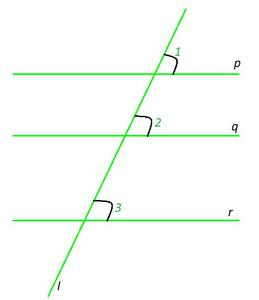Lines Parallel to the Same Line.

To Prove: Lines which are parallel to the same line are parallel to each other (p || q || r).

Proof: Line p and r are parallel and lines p and q are parallel to each other and line l is traversal.

Now, p || q and p || r

Since m || q therefore,

∠ 1 = ∠ 2                                                         (Corresponding angles axiom)                    ……(1)

∠ 1 = ∠ 3                                                         (Corresponding angles axiom)                    ……(2)

So, from equation (1) and (2) it is concluded that:

∠ 2 = ∠ 3

However, according to the converse of corresponding angles axiom, If a transversal intersects two lines such that a pair of corresponding angles are equal, then the two lines are parallel to each other.

Hence, the two lines q and r are parallel and so parallel to r.

### Sample Problems

Problem 1: In Figure, if PQ || RS, ∠ MXQ = 135° and ∠ MYR = 40°, find ∠ XMY.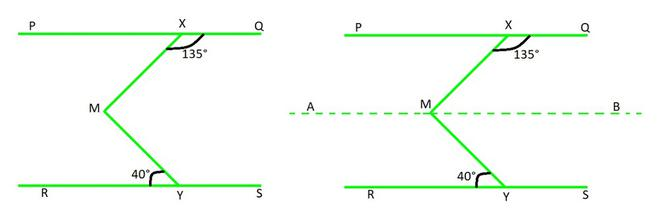Solution:

Lets construct a line AB parallel to line PQ, through point M.

Now, AB || PQ and PQ || RS

⇒ AB || RS || PQ (Theorem 5)

∠ QXM + ∠ XMB = 180°  (AB || PQ, Interior angles on the same side of the transversal XM)

As, ∠ QXM = 135°

135° + ∠ XMB = 180°

∠ XMB = 45°

Now, ∠ BMY = ∠ MYR (AB || RS, Alternate angles)

∠ BMY = 40°

As, ∠ XMB + ∠ BMY = 45° + 40°

Therefore, ∠ XMY = 85°.

Problem 2: If a transversal intersects two lines such that the bisectors of a pair of corresponding angles are parallel, then prove that the two lines are parallel.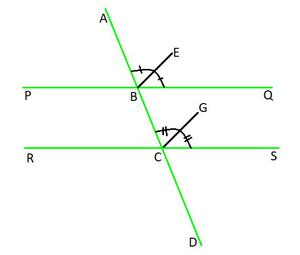Solution:

Let a transversal AD intersects two lines PQ and RS at points B and C respectively. Ray BE is the bisector of ∠ ABQ and ray CG is the bisector of ∠ BCS and BE || CG.

To prove: PQ || RS.

Given that, the ray BE is the bisector of ∠ ABQ.

Therefore, ∠ ABE = ½ ∠ ABQ                                                                        ……(1)

Similarly, ray CG is the bisector of ∠ BCS.

Therefore, ∠ BCG = ½ ∠ BCS                                                                      ……(2)

Using Corresponding angles axiom,

∠ ABE = ∠ BCG  (BE || CG and AD is the transversal)                                 ……(3)

Using (1) and (2) in (3), you get

½ ∠ ABQ = ½ ∠ BCS

That is, ∠ ABQ = ∠ BCS

As they are the corresponding angles formed by transversal AD with PQ and RS.

Therefore, PQ || RS.                                          (Converse of corresponding angles axiom)

Problem 3: In Figure, AB || CD and CD || EF. Also EA ⊥ AB. If ∠ BEF = 55°, find the values of x, y and z.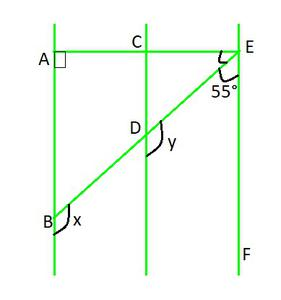Solution:

Since, AB || CD and CD || EF

⇒ AB || CD || EF            (Lines which are parallel to the same line are parallel to each other)

And, EB and AE are transversal.

y + 55° = 180°  (CD || EF, Interior angles on the same side of the transversal EB)

y = 180º – 55º = 125º

As, x = y (AB || CD, Corresponding angles axiom)

x = y = 125º

Now, ∠ EAB + ∠ FEA = 180° (Interior angles on the same side of the transversal EA)

90° + z + 55° = 180°

Hence, z = 35°.

Problem 4: In Figure, find the values of x and y and then show that AB || CD.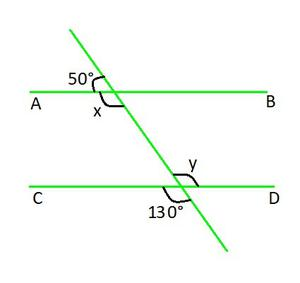Solution:

Here,

x+50° = 180° (linear pair is equal to 180°)

x = 130°

and, y = 130°  (vertically opposite angles are equal)

Here, what we can observe is,

x = y = 130°

In two parallel lines, the alternate interior angles are equal.

Hence, this proves that alternate interior angles are equal and so, AB || CD.

Problem 5: In Figure, if AB || CD, ∠ APQ = 50° and ∠ PRD = 127°, find x and y.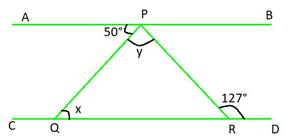Solution:

Here, APQ = PQR                                            (Alternate interior angles)

x = 50°

And,

APR = PRD                                                    (Alternate interior angles)

APQ+QPR = 127°

127° = 50°+ y

y = 77°

Hence, the values are, x = 50° and y = 77°.

Attention reader! All those who say programming isn’t for kids, just haven’t met the right mentors yet. Join the  Demo Class for First Step to Coding Coursespecifically designed for students of class 8 to 12.

The students will get to learn more about the world of programming in these free classes which will definitely help them in making a wise career choice in the future.

My Personal Notes arrow_drop_up